# DrumCube, an Arduino Robot Drummer

An Arduino-based robot drummer. It works with a transistor noise-generator and an arrangement of servos hitting a can and a pair of piezos.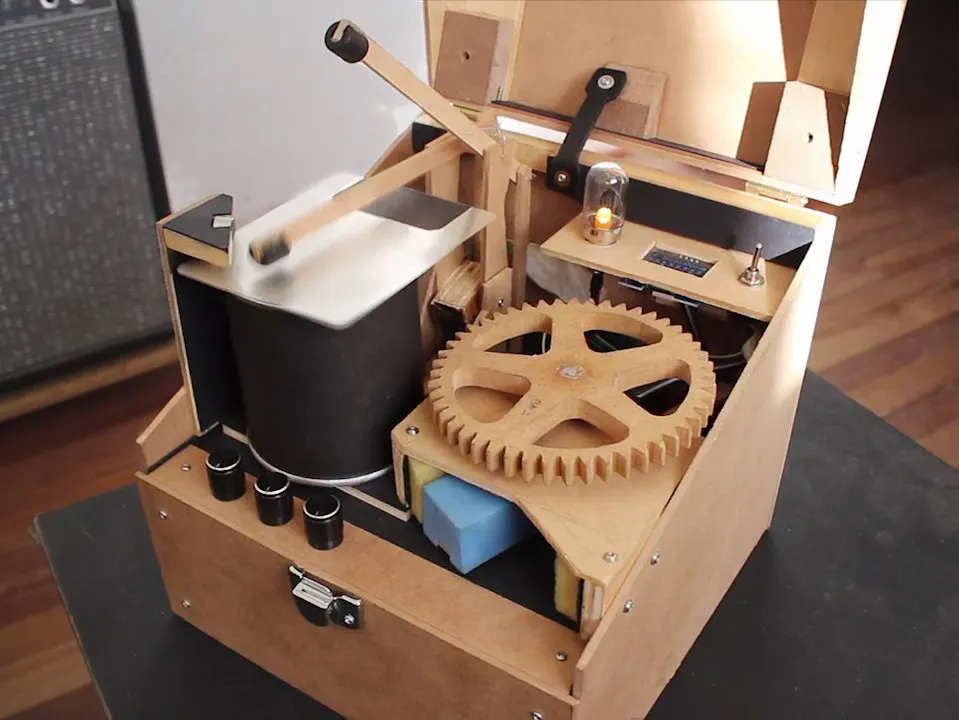## Things used in this project

### Hardware componentsArduino UNO & Genuino UNO
×1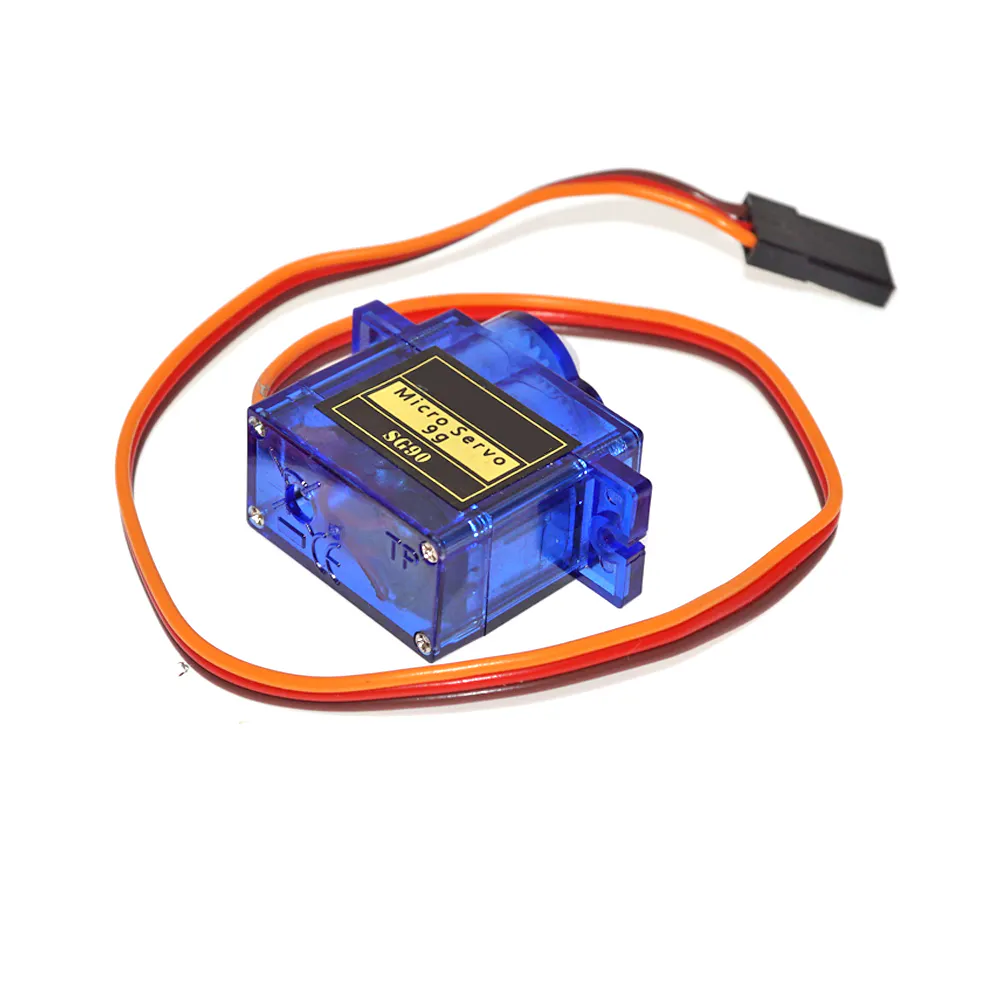SG90 Micro-servo motor
×3
 Ultra-small LM2596 Power Supply Module DC / DC BUCK 3A adjustable buck Module Regulator Ultra LM2596S Compatible With Arduino by Atomic Market step down module
×1
 LAOMAO DC-DC Step-up Boost Power Supply Module Adjustable Power Apply 3V-32V to 5V-35V XL6009 400KHz 4A Max Step up module
×1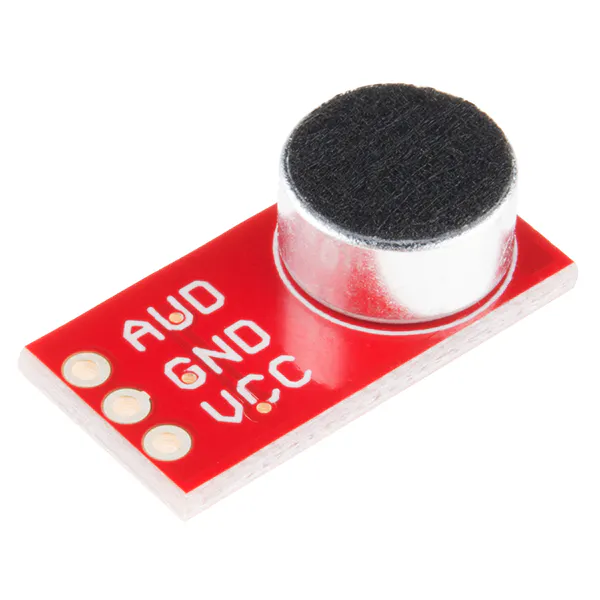SparkFun Electret Microphone Breakout
×1
 Piezo Element
×2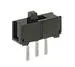Slide Switch
×1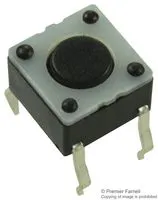Tactile Switch, Top Actuated
×1
 Transistor - NPN, 60V 200mA (2N3904)
×5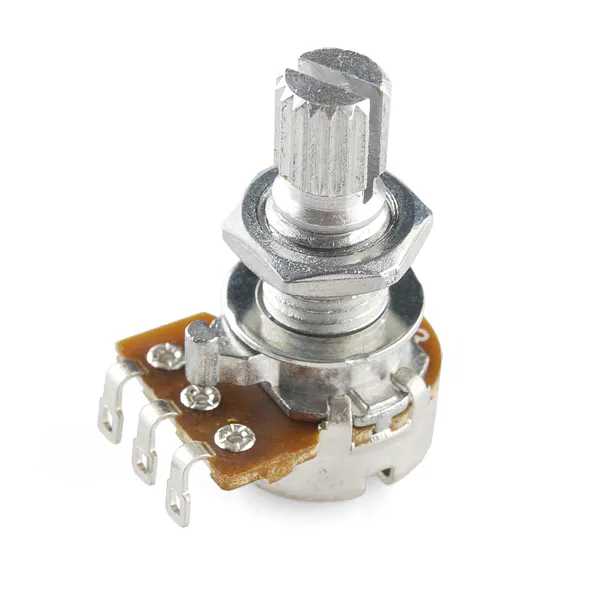Rotary potentiometer (generic) 500k
×3×1

### Software apps and online servicesArduino IDE

### Hand tools and fabrication machines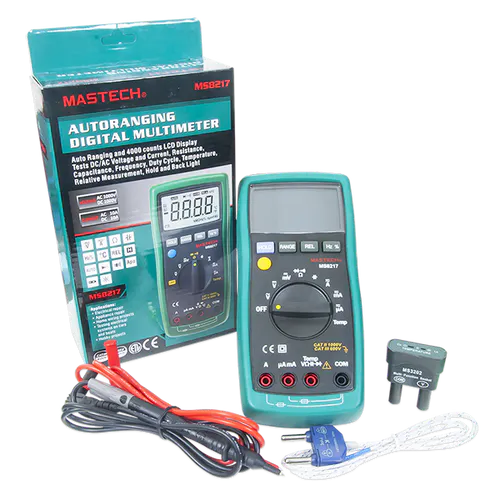Digilent Mastech MS8217 Autorange Digital MultimeterSoldering iron (generic)

## Schematics

### Full Diagram

Full diagram of the project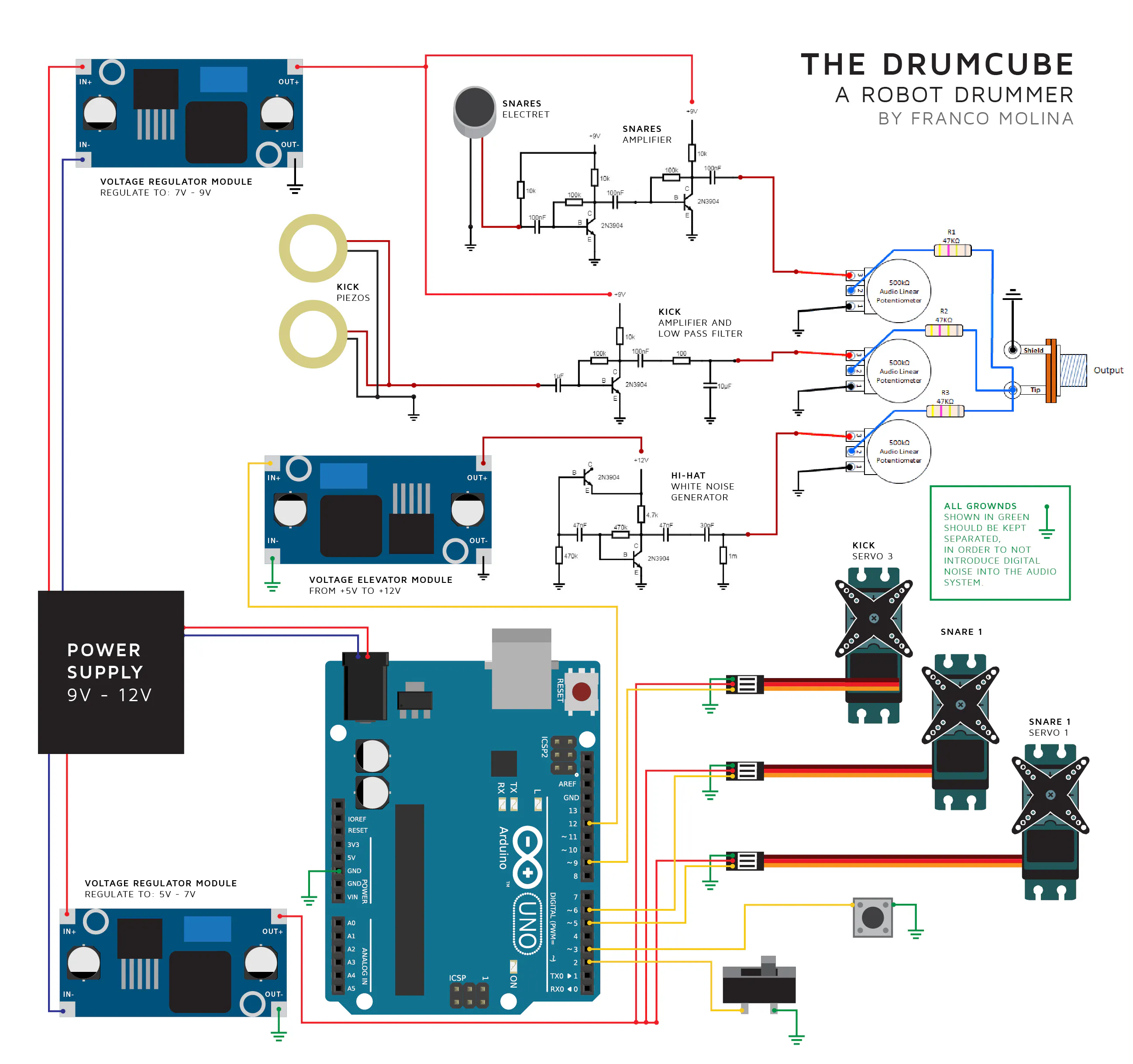### Kick Schematic

Amplifier circuit for the piezos in the kick system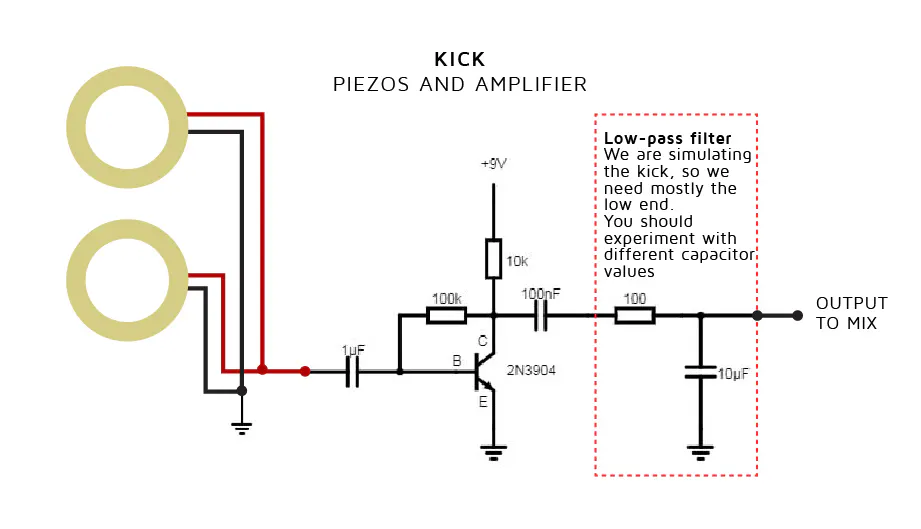### Snare Schematic

Amplifier circuit for the electret microphone in the Snare system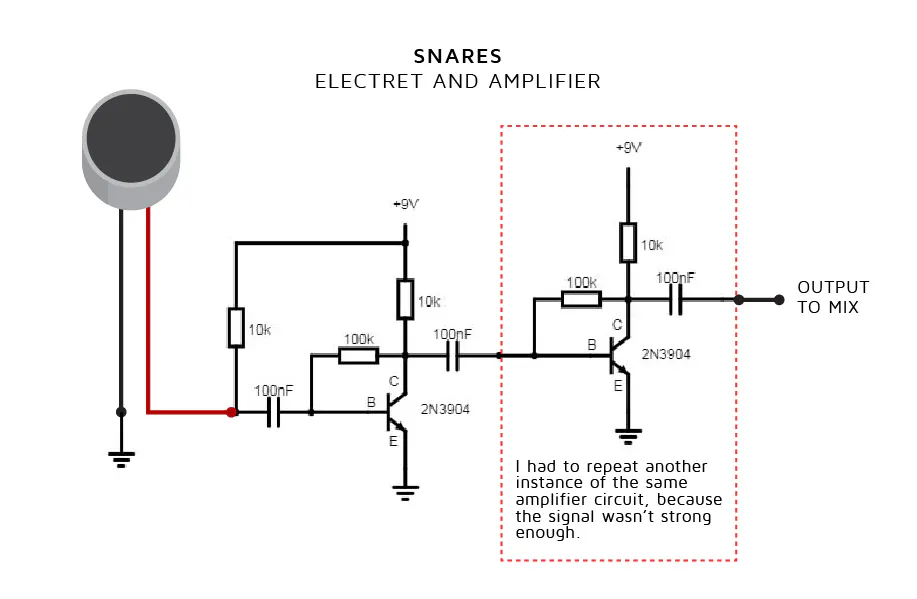### HiHat Generator Schematic

White noise generator and high-pass filter to replicate the cymbals sound.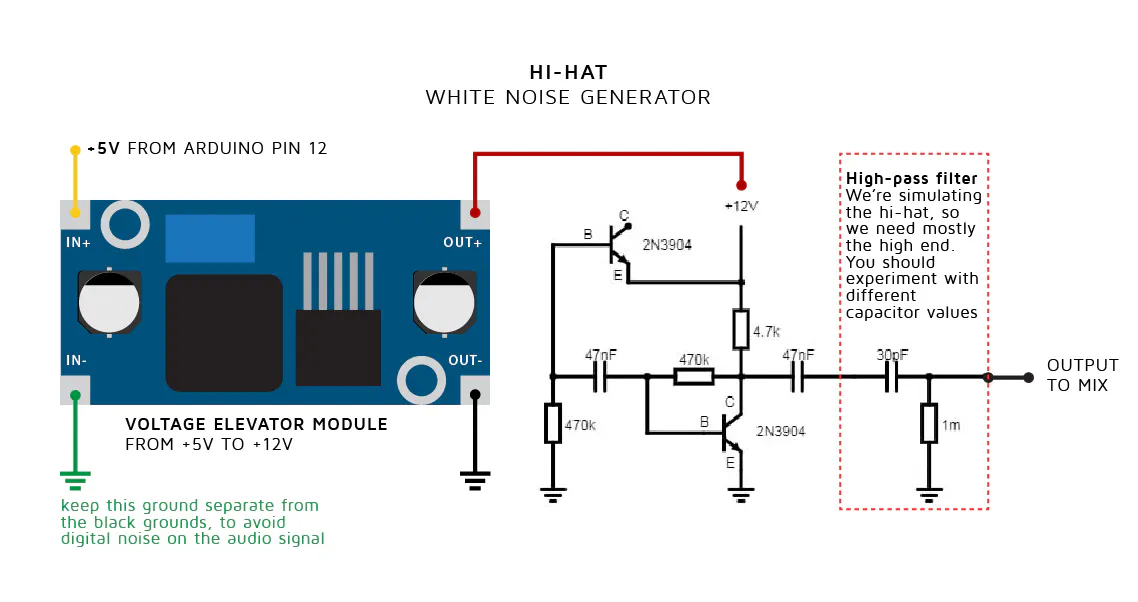### Audio signal mixer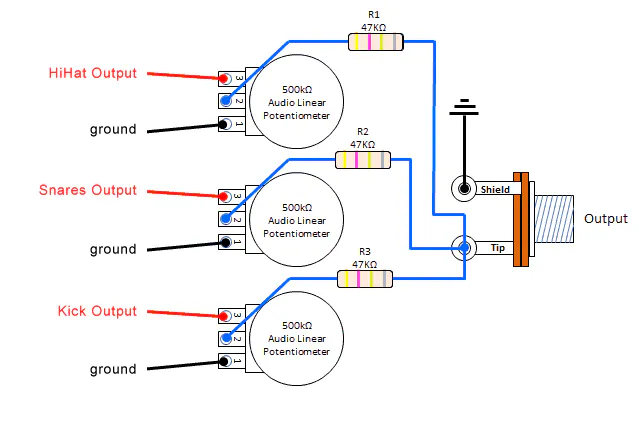## Code

### DrumCube, a robot drummer

Arduino
```// DrumCube - a robot drummer
// written by Franco Molina @artefrancomolina

// Setting servos
#include <Servo.h>
Servo servo1;
Servo servo2;
Servo servo3;

// Servo positions
// this will differ depending on your servos, please test yours to find out the values that best suit you
byte restServo1 = 12;
byte hitServo1 = 21;
byte restServo2 = 123;
byte hitServo2 = 114;
byte restServo3 = 4;
byte hitServo3 = 19;

// Setting the HiHat white noise generator
#define noiseGenerator 12
#define noiseLed 13 //turn on led as a visual representation of the noise being generated

// Setting servo times
// this will also differ depending on your servos, please test yours to find out the values that best suit you
byte timeSnare1 = 110;
byte timeSnare2 = 108;
byte timeKick = 71;
byte timeHihat = 20;
byte sustainTimeHihat = 70;

// Setting previous Snare and Kick
byte previousSnare = 2;
byte previousKick = 0;

// Setting cycle times
static unsigned long timeStartCycle;
static unsigned long timeTotalCycle; // changing this, change how fast/slow the rhythm is, you can set a specific value for each drumbeat in the selectDrumBeat() function

// Bar and Cycles variables
int cycleNumber;
int cycleNumbers[] = {6, 0, 5, 0, 8, 0, 5, 0, 6, 0, 5, 0, 8, 0, 5, 0}; // this array stores the drum elements combination for each cycle (semiquaver or sixteenth) of the drumbeat
byte timeSignature = 44;
int drumbeatID = 0; // ID number for each drumbeat

//
int i; // for counting the time in the "for loop" of the cyclePlayer function
int b; // for the "for loop" that counts the number of cycles

// control interface
#define switchPlayStop 2  // switch bettween playing and stop
#define buttonSelect 3    // tact button that select the drumbeat to play

void setup(void) {

// define pins for each servo
pinMode (5, OUTPUT);
pinMode (6, OUTPUT);
pinMode (9, OUTPUT);

// attach servos to those pins
servo1.attach(5); //caja 1
servo2.attach(6); //caja 2
servo3.attach(9); //bombo
delay(150);

// put all servos on rest position
servo1.write(restServo1);
servo2.write(restServo2);
servo3.write(restServo3);
delay(300);

// Setting hihat and led pinmodes
pinMode (noiseGenerator, OUTPUT);
digitalWrite(noiseGenerator, LOW);
pinMode (noiseLed, OUTPUT);
digitalWrite(noiseLed, LOW);

// Setting control interface pinmodes
pinMode (switchPlayStop, INPUT_PULLUP);
pinMode (buttonSelect, INPUT_PULLUP);

}

void loop(void) {
if (switchPlayStop == LOW) { //if the play/stop switch is ON (logical LOW)

//We start a "for loop" to play a semiquaver cycle a maximum of 16 times, for every bar
for (b = 0; b < 16; b = b + 1) {

selectDrumBeat(); // select the drumbeat based on the drumbeatID selected
cyclePlayer(cycleNumbers[b]); //play the semiquaver cycle for each number stored in the cycleNumbers array

// if we reach the maximum number of cycles (16 for 4/4, 12 for 6/8, 12/8 or 3/4) we start again
if (b == 11 && (timeSignature == 68 || timeSignature == 128)) {
b = -1;
}
if (b == 15) {
b = -1;
}
}

}
// If the play/stop switch is OFF (logical HIGH) we enter settingMode
else {
settingMode(); // function that lets you choose between different drumbeats and wait for the play switch to be activated
}
}

// CYCLE PLAYER
// this functions runs a semiquaver cycle every time is called.
void cyclePlayer(int cycleNumber) { // we store every single value of the cycleNumbers array into the cycleNumber variable
timeStartCycle = millis(); // we save the starting time, to compare it later with the actual time and get the time past

//set both snare servos to rest position in case they were on hit position
servo1.write(restServo1);
servo2.write(restServo2);

//we star a "for loop" for the entire duration of every semiquaver cycle
for (i = 0; i < timeTotalCycle; i++) {

//now we send the hitting commands on time so they reach their destination on time (the end of the semiquaver cycle)

// if we reach the time to send the command to the snare on servo1. We start checking the element that takes the longest time to move, in this case is servo1, in yours could be different.
if ((millis() - timeStartCycle >= timeTotalCycle - timeSnare1)) {
// we check if this is the one snare of the two, that has to be played,
// and also, if in this cycle a snare has to be played at, all based on the cycleNumber number (this number define the cobination of drum elements)
if ((previousSnare == 2) && ((cycleNumber == 9) || (cycleNumber == 8) || (cycleNumber == 4) || (cycleNumber == 3)) ) {
servo1.write(hitServo1 + 5); // we send the servo command
}
// if we reach the time to send the command to the snare on servo2
if (millis() - timeStartCycle >= timeTotalCycle - timeSnare2) {
// we check if this is the one snare of the two, that has to be played, and also if in this cycle a snare has to be played at all
if ((previousSnare == 1) && ((cycleNumber == 9) || (cycleNumber == 8) || (cycleNumber == 4) || (cycleNumber == 3)) ) {
servo2.write(hitServo2 - 5); // we send the servo command
}
// we now check if we reached the time to send the command to the third servo, the kick
if ((millis() - timeStartCycle >= timeTotalCycle - timeKick)) {
// we check if in this cycle a snare has to be played
if ( (cycleNumber == 9) || (cycleNumber == 4) || (cycleNumber == 6) || (cycleNumber == 1) ) {
// we check on what position was previosly the servo, either hiting the left or the right piezo, this state will be saved later
if (previousKick == 0) {
servo3.write(hitServo3);
}
else if (previousKick == 1) {
servo3.write(restServo3);
}
}
// finally, we check if the time to turn on the white noise generator was reached, for the hihat
if (millis() - timeStartCycle >= (timeTotalCycle - timeHihat)) {
// we check if in this cycle the hihat has to be played
if ( (cycleNumber == 9) || (cycleNumber == 8) || (cycleNumber == 6) || (cycleNumber == 5) ) {
digitalWrite(noiseLed, HIGH);
digitalWrite(noiseGenerator, HIGH);
}
}
}
}
}
// This is where the semiquaver cycle ends.

// HIHAT DURATION
// turn off hi-hat noise generator, but only under the following conditions:
//    //if the noise-generator was ON        //the time past exceed the time set as sustain          //the time past does not reach yet the point where a new noise could start
if ( (digitalRead(noiseGenerator) == HIGH) && (millis() - timeStartCycle >= sustainTimeHihat) && (millis() - timeStartCycle < (timeTotalCycle - (timeHihat + 10))) ) {
digitalWrite(noiseGenerator, LOW);
digitalWrite(noiseLed, LOW);
}

// Check if the play/stop switch is switched OFF
i = timeTotalCycle;                //reset time counting
digitalWrite(noiseGenerator, LOW);  //turn off noise
digitalWrite(noiseLed, LOW);        //turn off noise
servo1.write(restServo1);           //stop servos
servo2.write(restServo2);           //stop servos
}

delay(1);
}

// If one of the Snares was hit, then declare it as the previous one, in order to hit the other one the next time
if ((cycleNumber == 9) || (cycleNumber == 8) || (cycleNumber == 4) || (cycleNumber == 3)) {
switch (previousSnare) {
case 1:
previousSnare = 2;
break;
case 2:
previousSnare = 1;
break;
default:
break;
}
}

// If one of the piezo kick was hit, then declare it as the previous one, in order to hit the other one the next time
if ( (cycleNumber == 9) || (cycleNumber == 4) || (cycleNumber == 6) || (cycleNumber == 1) ) {
switch (previousKick) {
case 0:
previousKick = 1;
break;
case 1:
previousKick = 0;
break;
default:
break;
}
}
}

// SETTING MODE
// this mode is activated when the play/stop switch is swiched OFF (logical LOW)
void settingMode() {
while (digitalRead(switchPlayStop) == HIGH) { // keep doing the following actions while the play/stop switch is OFF
if (digitalRead(buttonSelect) == LOW) { // if the selection button is pressed (logical LOW), we change the drumbeat
drumbeatID ++; // we select the next drumbeat each time the button is pressed
selectDrumBeat(); // select the drumbeat based on the drumbeatID
// OPTIONAL
// Here you can add whatever piece of code to indicate which drumbeat is being selected,
// this could be turning in a led, sending a serial.print(), etc.
if (drumbeatID > 7) { //in case we exceed the total number of drumbeats availabe (), we go back to 0.
drumbeatID = 0;
}
delay(100); //delay to avoid detecting button twice when pressed once
}
}
}

// SELECT DRUMBEAT
// this functions uses the value stored in drumbeatID, to modify all the variables needed in order to choose between drumbeats.
void selectDrumBeat() {
switch (drumbeatID) {
case 1: // DiscoBasic
timeTotalCycle = 124;
timeSignature = 44;
cycleNumbers = 1;
cycleNumbers = 0;
cycleNumbers = 5;
cycleNumbers = 0;
cycleNumbers = 4;
cycleNumbers = 0;
cycleNumbers = 5;
cycleNumbers = 0;
cycleNumbers = 1;
cycleNumbers = 0;
cycleNumbers = 5;
cycleNumbers = 0;
cycleNumbers = 4;
cycleNumbers = 0;
cycleNumbers = 5;
cycleNumbers = 0;
break;
case 2: // NewBugalú
timeTotalCycle = 155;
timeSignature = 44;
cycleNumbers = 6;
cycleNumbers = 0;
cycleNumbers = 5;
cycleNumbers = 0;
cycleNumbers = 8;
cycleNumbers = 0;
cycleNumbers = 5;
cycleNumbers = 3;
cycleNumbers = 5;
cycleNumbers = 3;
cycleNumbers = 6;
cycleNumbers = 0;
cycleNumbers = 8;
cycleNumbers = 0;
cycleNumbers = 5;
cycleNumbers = 0;
break;
case 3: // HiHat16
timeTotalCycle = 134;
timeSignature = 44;
cycleNumbers = 6;
cycleNumbers = 5;
cycleNumbers = 5;
cycleNumbers = 5;
cycleNumbers = 8;
cycleNumbers = 5;
cycleNumbers = 5;
cycleNumbers = 5;
cycleNumbers = 6;
cycleNumbers = 5;
cycleNumbers = 5;
cycleNumbers = 5;
cycleNumbers = 8;
cycleNumbers = 5;
cycleNumbers = 5;
cycleNumbers = 5;
break;
case 4: // SwingGroove
timeTotalCycle = 153;
timeSignature = 128; // (12/8)
cycleNumbers = 6;
cycleNumbers = 0;
cycleNumbers = 0;
cycleNumbers = 6;
cycleNumbers = 0;
cycleNumbers = 5;
cycleNumbers = 6;
cycleNumbers = 0;
cycleNumbers = 0;
cycleNumbers = 6;
cycleNumbers = 0;
cycleNumbers = 5;
break;
case 5: // BossaNova
timeTotalCycle = 200;
timeSignature = 44;
cycleNumbers = 6;
cycleNumbers = 5;
cycleNumbers = 5;
cycleNumbers = 9;
cycleNumbers = 6;
cycleNumbers = 5;
cycleNumbers = 8;
cycleNumbers = 6;
cycleNumbers = 6;
cycleNumbers = 5;
cycleNumbers = 8;
cycleNumbers = 6;
cycleNumbers = 9;
cycleNumbers = 5;
cycleNumbers = 5;
cycleNumbers = 6;
break;
case 6: // ShuffleGroove
timeTotalCycle = 134;
timeSignature = 128; // (12/8)
cycleNumbers = 6;
cycleNumbers = 0;
cycleNumbers = 5;
cycleNumbers = 9;
cycleNumbers = 0;
cycleNumbers = 5;
cycleNumbers = 6;
cycleNumbers = 0;
cycleNumbers = 5;
cycleNumbers = 9;
cycleNumbers = 0;
cycleNumbers = 5;
break;
case 7: // Franco's beat
timeTotalCycle = 142;
timeSignature = 44;
cycleNumbers = 6;
cycleNumbers = 0;
cycleNumbers = 5;
cycleNumbers = 1;
cycleNumbers = 8;
cycleNumbers = 0;
cycleNumbers = 6;
cycleNumbers = 0;
cycleNumbers = 5;
cycleNumbers = 1;
cycleNumbers = 6;
cycleNumbers = 0;
cycleNumbers = 8;
cycleNumbers = 0;
cycleNumbers = 6;
cycleNumbers = 0;
break;
default: // Basic 4/4
timeTotalCycle = 144;
timeSignature = 44;
cycleNumbers = 6;
cycleNumbers = 0;
cycleNumbers = 5;
cycleNumbers = 0;
cycleNumbers = 8;
cycleNumbers = 0;
cycleNumbers = 5;
cycleNumbers = 0;
cycleNumbers = 6;
cycleNumbers = 0;
cycleNumbers = 5;
cycleNumbers = 0;
cycleNumbers = 8;
cycleNumbers = 0;
cycleNumbers = 5;
cycleNumbers = 0;
break;
}
}
```

## Credits

### FrancoMolina

1 project • 15 followers